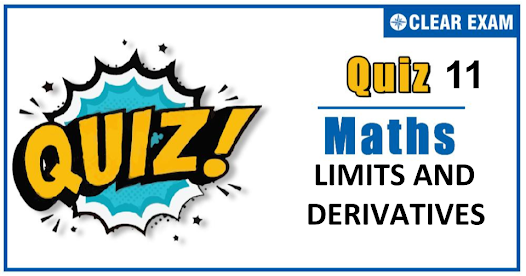## LIMITS AND DERIVATIVES quiz-11

As per analysis for previous years, it has been observed that students preparing for JEE MAINS find Mathematics out of all the sections to be complex to handle and the majority of them are not able to comprehend the reason behind it. This problem arises especially because these aspirants appearing for the examination are more inclined to have a keen interest in Mathematics due to their ENGINEERING background. Furthermore, sections such as Mathematics are dominantly based on theories, laws, numerical in comparison to a section of Engineering which is more of fact-based,physics and includes substantial explanations. By using the table given below, you easily and directly access to the topics and respective links of MCQs. Moreover, to make learning smooth and efficient, all the questions come with their supportive solutions to make utilization of time even more productive. Students will be covered for all their studies as the topics are available from basics to even the most advanced. .

Q1. limx→0⁡{(1x+2x+3x+⋯+nx)/n}(1/x) is equal to
•  (n!)n
•  (n!)1/n
•  n!
•  ln ⁡n!
Solution
We have, limx→0⁡{(1x+2x+⋯+nx)/n}(1/x) =limx→0⁡{1+(1x-1)/n+(2x-1)/n+⋯+(nx-1)/n}(1/x) =elimx→01/n {(1x-1)/n+(2x-1)/n+⋯+(nx-1)/n} =e1/n [log⁡1+log⁡2+⋯+log⁡n ] =e1/n(log⁡n!)=elog⁡(n!)(1/n) =(n!)1/n

Q2.The value of limx→∞⁡(√(1+x4 )-(1+x2))/x2 is equal to
•  0
•  -1
•  2
•  None of these
Solution
limx→∞(⁡(√(1+x4 )-(1+x2))/x2 =limx→∞ (√(1+1/x4 )-(1+1/x2 ))/1 =(1-1)/1=0

Q3. limx→π/6⁡(2 sin2⁡x+sin⁡x-1 )/(2 sin2⁡x-3 sin⁡x+1 ) is equal to
•   3
•  -3
•   6
•   0
Solution
limx→π/6⁡(2 sin2⁡x+sin⁡x-1 )/(2 sin2⁡x-3 sin⁡x+1 ) =limx→π/6)⁡(4 sin⁡x cos⁡x+cos⁡x)/(4 sin⁡x cos⁡x-3 cos⁡x ) [by L’Hospital’s rule] =limx→π/6⁡(cos⁡x (4 sin⁡x+1))/(cos⁡x (4 sin⁡x-3)) =(4 sin⁡π/6+1)/(4 sin⁡π/6-3 )=-3

Q4. The value of limn→∞⁡{1/3+2/21+3/91+⋯+n/(n4+n2+1)}, is
•  1
•  1/2
•  1/3
•  None of these
Solution
We have, {1/3+2/21+3/91+⋯+n/(n4+n2+1)} =∑nr=1 r/(r4+r2+1) =∑nr=1 r/((r2+1)2-r2 ) =1/2 ∑nr=1 2r/((r2+r+1)(r2-r+1)) =1/2 ∑nr=1 {1/(r2-r+1)-1/(r2+r+1)} =1/2 {(1/1-1/3)+(1/3-1/7)+(1/7-1/13)+⋯+(1/(n2-n+1)-1/(n2+n+1)) } =1/2 (1-1/(n2+n+1))=(n2+n)/(2(n2+n+1)) ∴ Requires limit =limn→∞(n2+n)/(2(n2+n+1))=1/2

Q5.The value of limx→∞⁡√(a2 x2+ax+1)-√(a2 x2+1)〗 is
•  1/2
•  1
•  2
•  None of these
Solution
limx→∞⁡√(a2 x2+ax+1)-√(a2 x2+1) =limx→∞⁡(a2 x2+ax+1-a2 x2-1)/(√(a2 x2+ax+1)+√(a2 x2+1)) =limx→∞⁡ax/x[√(a2+a/x+1/x2 )+√(a2+1/x2 )] =a/(√(a2 )+√(a2 ))=1/2

Q6. limx→π/4⁡(∫2sec2⁡x f(t)dt)/(x22/16) equals
•  8/π f(2)
•  2/π f(2)
• 2/π f(1/2)
•  4f(2)
Solution
limx→π/4⁡(∫2sec2⁡x f(t)dt)/(x22/16) =limx→π/4 (2 sec2⁡x tan⁡x f(sec2⁡x))/2x [Using Leibniz and L’ Hospital’s rules] =(sec2⁡π/2 f(sec2⁡π/4 ) tan⁡π/4)/(π/4)=8/π f(2)

Q7.Let f(x) be twice differentiable function such that f'' (0)=2. Then, limx→0⁡(2f(x)-3f(2x)+f(4x))/x2 is
•  6
•  3
•  12
•  None of these
Solution
We have, limx→0⁡(2f(x)-3f(2x)+f(4x))/x2 [0/0 form] =limx→0⁡(2f' (x)-6f' (2x)+4f' (4x))/2 [Using L’ Hospital’s Rule] =limx→0⁡(2f'' (x)12f'' (2x)+16f'' (4x))/2 [Using L’ Hospital’s Rule] =(2 f"(0)-12f" (0)+16f" (0))/2 =3f'' (0)=3×2=6

Q8. If f:R→R is defined by f(x)={ ((x-2)/(x2-3x+2),if x∈R-{1,2} 2, if x=1 1, if x=2) Then lim(x→2)⁡(f(x)-f(2))/(x-2) is equal to
•  0
•  -1
•  1
•  -1/2
Solution
limx→2⁡(f(x)-f(2))/(x-2)=limx→2⁡((x-2)/(x2-3x+2)-1)/(x-2) =limx→2 (x-2-(x2-3x+2))/((x-2)(x2-3x+2)) =limx→2 (-(x-2)2)/((x-2)(x-2)(x-1)) =-limx→2 1/(x-1) =-1

Q9.limx→0⁡(ax-bx)/(ex-1) is equal to
•  loge⁡(a/b)
•  loge⁡(b/a)
•  loge⁡(ab)
•  loge⁡(a+b)
Solution
limx→0⁡(ax-bx)/(ex-1)=limx→0⁡(ax-bx)/x.x/(ax-1) =[limx→0 ((ax-1)/x)-limx→0 ((bx-1)/x) ].limx→0 x/(ex-1) =(loge⁡a-loge⁡b ).limx→0 1/((ex-1)/x) =loge⁡(a/b)

Q10. The value of limx→∞⁡((3x-4)/(3x+4))((x+1)/3) ) , is
•  e-2/3
•  e-1/3
•  e-2
•  e-1
Solution
We have, limx→∞⁡((3x-4)/(3x+4))((x+1)/3) ) =limx→∞⁡(1+(-6)/(3x+2))((x+1)/2) =elimx→∞⁡(-6)/(3x+2)×(x+1)/3 =elimx→∞⁡(-2x-2)/(3x+2) =e(-2/3)## Want to know more

Please fill in the details below:

## Latest NEET Articles\$type=three\$c=3\$author=hide\$comment=hide\$rm=hide\$date=hide\$snippet=hide

Name

ltr
item
BEST NEET COACHING CENTER | BEST IIT JEE COACHING INSTITUTE | BEST NEET & IIT JEE COACHING: QUIZ-11 LIMITS AND DERIVATIVES
QUIZ-11 LIMITS AND DERIVATIVES
https://1.bp.blogspot.com/-z3pUxpTf9bI/X8Hl6PA4nUI/AAAAAAAAEyU/y9XXUT6t5KQmvUOnSle1kV53n9UI4JkjQCLcBGAsYHQ/w599-h276/Quiz%2BImage%2B20%2B%252823%2529.png
https://1.bp.blogspot.com/-z3pUxpTf9bI/X8Hl6PA4nUI/AAAAAAAAEyU/y9XXUT6t5KQmvUOnSle1kV53n9UI4JkjQCLcBGAsYHQ/s72-w599-c-h276/Quiz%2BImage%2B20%2B%252823%2529.png
BEST NEET COACHING CENTER | BEST IIT JEE COACHING INSTITUTE | BEST NEET & IIT JEE COACHING
https://www.cleariitmedical.com/2020/11/QUIZ-11%20LIMITS%20AND%20DERIVATIVES.html
https://www.cleariitmedical.com/
https://www.cleariitmedical.com/
https://www.cleariitmedical.com/2020/11/QUIZ-11%20LIMITS%20AND%20DERIVATIVES.html
true
7783647550433378923
UTF-8

STAY CONNECTED